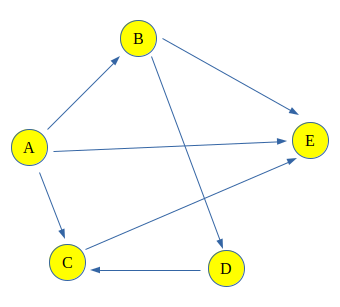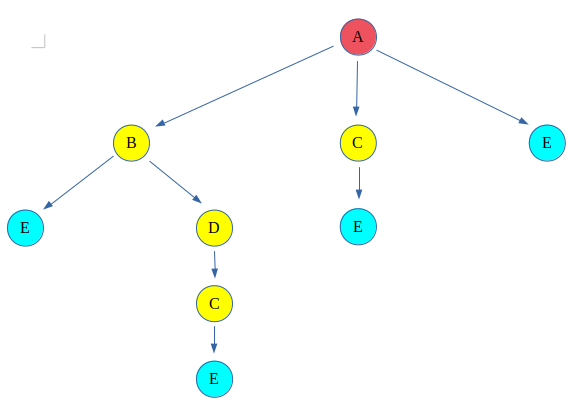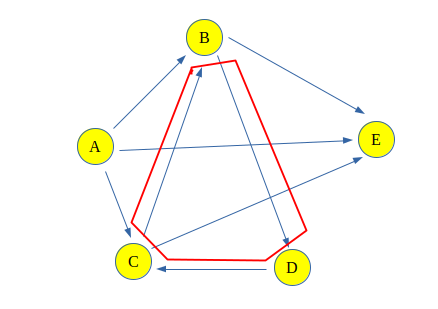# Count all possible paths between two vertices

• Difficulty Level : Medium
• Last Updated : 23 Nov, 2021

Count the total number of ways or paths that exist between two vertices in a directed graph. These paths don’t contain a cycle, the simple enough reason is that a cycle contains an infinite number of paths and hence they create a problem.
Examples:

`For the following Graph:````
Input: Count paths between A and E
Output : Total paths between A and E are 4
Explanation: The 4 paths between A and E are:
A -> E
A -> B -> E
A -> C -> E
A -> B -> D -> C -> E

Input : Count paths between A and C
Output : Total paths between A and C are 2
Explanation: The 2 paths between A and C are:
A -> C
A -> B -> D -> C```

Approach:
The problem can be solved using backtracking, that says take a path and start walking on it and check if it leads us to the destination vertex then count the path and backtrack to take another path. If the path doesn’t lead to the destination vertex, discard the path.
This type of graph traversal is called Backtracking.
Backtracking for above graph can be shown like this:
The red color vertex is the source vertex and the light-blue color vertex is destination, rest are either intermediate or discarded paths.This give four paths between source(A) and destination(E) vertex.
Why this solution will not work for a graph which contains cycles?
The Problem Associated with this is that now if one more edge is added between C and B, it would make a cycle (B -> D -> C -> B). And hence after every cycle through the loop, the length path will increase and that will be considered as a different path, and there would be infinitely many paths because of the cycle.Algorithm:

1. Create a recursive function that takes index of node of a graph and the destination index. Keep a global or a static variable count to store the count. Keep a record of the nodes visited in the current path by passing a visited array by value (instead of reference, which would not be limited to the current path).
2. If the current nodes is the destination increase the count.
3. Else for all the adjacent nodes, i.e. nodes that are accessible from the current node, call the recursive function with the index of adjacent node and the destination.
4. Print the Count.

Implementation:

## C++

 `/*`` ``* C++ program to count all paths from a source to a`` ``* destination.`` ``* https://www.geeksforgeeks.org/count-possible-paths-two-vertices/`` ``* Note that the original example has been refactored.`` ``*/``#include ``#include ``#include ``#include ``using` `namespace` `std;` `/*`` ``* A directed graph using adjacency list representation;`` ``* every vertex holds a list of all neighbouring vertices`` ``* that can be reached from it.`` ``*/``class` `Graph {``public``:``    ``// Construct the graph given the number of vertices...``    ``Graph(``int` `vertices);``    ``// Specify an edge between two vertices``    ``void` `add_edge(``int` `src, ``int` `dst);``    ``// Call the recursive helper function to count all the``    ``// paths``    ``int` `count_paths(``int` `src, ``int` `dst, ``int` `vertices);` `private``:``    ``int` `m_vertices;``    ``list<``int``>* m_neighbours;``    ``void` `path_counter(``int` `src, ``int` `dst, ``int``& path_count,``                      ``vector<``bool``>& visited);``};` `Graph::Graph(``int` `vertices)``{``    ``m_vertices = vertices; ``// unused!!``    ``/* An array of linked lists - each element corresponds``    ``to a vertex and will hold a list of neighbours...*/``    ``m_neighbours = ``new` `list<``int``>[vertices];``}` `void` `Graph::add_edge(``int` `src, ``int` `dst)``{``    ``m_neighbours[src].push_back(dst);``}` `int` `Graph::count_paths(``int` `src, ``int` `dst, ``int` `vertices)``{``    ``int` `path_count = 0;``    ``vector<``bool``> visited(vertices, ``false``);``    ``path_counter(src, dst, path_count, visited);``    ``return` `path_count;``}` `/*`` ``* A recursive function that counts all paths from src to`` ``* dst. Keep track of the count in the parameter.`` ``*/``void` `Graph::path_counter(``int` `src, ``int` `dst, ``int``& path_count,``                         ``vector<``bool``>& visited)``{``    ``// If we've reached the destination, then increment``    ``// count...``    ``visited[src] = ``true``;``    ``if` `(src == dst) {``        ``path_count++;``    ``}``    ``// ...otherwise recurse into all neighbours...``    ``else` `{``        ``for` `(``auto` `neighbour : m_neighbours[src]) {``            ``if` `(!visited[neighbour])``                ``path_counter(neighbour, dst, path_count,``                             ``visited);``        ``}``    ``}``  ``visited[src] = ``false``;``}` `// Tests...``int` `main()``{``    ``// Create a graph given in the above diagram - see link``    ``Graph g(5);``    ``g.add_edge(0, 1);``    ``g.add_edge(0, 2);``    ``g.add_edge(0, 4);``    ``g.add_edge(1, 3);``    ``g.add_edge(1, 4);``    ``g.add_edge(2, 3);``    ``g.add_edge(2, 1);``    ``g.add_edge(3, 2);``    ``// Validate it...``    ``cout << g.count_paths(0, 4, 5);` `    ``return` `0;``}`

## Java

 `// Java program to count all paths from a source``// to a destination.``import` `java.util.Arrays;``import` `java.util.Iterator;``import` `java.util.LinkedList;` `// This class represents a directed graph using``// adjacency list representation` `class` `Graph {` `    ``// No. of vertices``    ``private` `int` `V;` `    ``// Array of lists for``    ``// Adjacency List``    ``// Representation``    ``private` `LinkedList adj[];` `    ``@SuppressWarnings``(``"unchecked"``) Graph(``int` `v)``    ``{``        ``V = v;``        ``adj = ``new` `LinkedList[v];``        ``for` `(``int` `i = ``0``; i < v; ++i)``            ``adj[i] = ``new` `LinkedList<>();``    ``}` `    ``// Method to add an edge into the graph``    ``void` `addEdge(``int` `v, ``int` `w)``    ``{` `        ``// Add w to v's list.``        ``adj[v].add(w);``    ``}` `    ``// A recursive method to count``    ``// all paths from 'u' to 'd'.``    ``int` `countPathsUtil(``int` `u, ``int` `d, ``int` `pathCount)``    ``{` `        ``// If current vertex is same as``        ``// destination, then increment count``        ``if` `(u == d) {``            ``pathCount++;``        ``}` `        ``// Recur for all the vertices``        ``// adjacent to this vertex``        ``else` `{``            ``Iterator i = adj[u].listIterator();``            ``while` `(i.hasNext()) {``                ``int` `n = i.next();``                ``pathCount = countPathsUtil(n, d, pathCount);``            ``}``        ``}``        ``return` `pathCount;``    ``}` `    ``// Returns count of``    ``// paths from 's' to 'd'``    ``int` `countPaths(``int` `s, ``int` `d)``    ``{` `        ``// Call the recursive method``        ``// to count all paths``        ``int` `pathCount = ``0``;``        ``pathCount = countPathsUtil(s, d, pathCount);``        ``return` `pathCount;``    ``}` `    ``// Driver Code``    ``public` `static` `void` `main(String args[])``    ``{``        ``Graph g = ``new` `Graph(``5``);``        ``g.addEdge(``0``, ``1``);``        ``g.addEdge(``0``, ``2``);``        ``g.addEdge(``0``, ``3``);``        ``g.addEdge(``1``, ``3``);``        ``g.addEdge(``2``, ``3``);``        ``g.addEdge(``1``, ``4``);``        ``g.addEdge(``2``, ``4``);` `        ``int` `s = ``0``, d = ``3``;``        ``System.out.println(g.countPaths(s, d));``    ``}``}` `// This code is contributed by shubhamjd.`

## Python3

 `# Python 3 program to count all paths``# from a source to a destination.` `# A directed graph using adjacency``# list representation`  `class` `Graph:` `    ``def` `__init__(``self``, V):``        ``self``.V ``=` `V``        ``self``.adj ``=` `[[] ``for` `i ``in` `range``(V)]` `    ``def` `addEdge(``self``, u, v):` `        ``# Add v to u’s list.``        ``self``.adj[u].append(v)` `    ``# Returns count of paths from 's' to 'd'``    ``def` `countPaths(``self``, s, d):` `        ``# Mark all the vertices``        ``# as not visited``        ``visited ``=` `[``False``] ``*` `self``.V` `        ``# Call the recursive helper``        ``# function to print all paths``        ``pathCount ``=` `[``0``]``        ``self``.countPathsUtil(s, d, visited, pathCount)``        ``return` `pathCount[``0``]` `    ``# A recursive function to print all paths``    ``# from 'u' to 'd'. visited[] keeps track``    ``# of vertices in current path. path[]``    ``# stores actual vertices and path_index``    ``# is current index in path[]``    ``def` `countPathsUtil(``self``, u, d,``                       ``visited, pathCount):``        ``visited[u] ``=` `True` `        ``# If current vertex is same as``        ``# destination, then increment count``        ``if` `(u ``=``=` `d):``            ``pathCount[``0``] ``+``=` `1` `        ``# If current vertex is not destination``        ``else``:` `            ``# Recur for all the vertices``            ``# adjacent to current vertex``            ``i ``=` `0``            ``while` `i < ``len``(``self``.adj[u]):``                ``if` `(``not` `visited[``self``.adj[u][i]]):``                    ``self``.countPathsUtil(``self``.adj[u][i], d,``                                        ``visited, pathCount)``                ``i ``+``=` `1` `        ``visited[u] ``=` `False`  `# Driver Code``if` `__name__ ``=``=` `'__main__'``:` `    ``# Create a graph given in the``    ``# above diagram``    ``g ``=` `Graph(``4``)``    ``g.addEdge(``0``, ``1``)``    ``g.addEdge(``0``, ``2``)``    ``g.addEdge(``0``, ``3``)``    ``g.addEdge(``2``, ``0``)``    ``g.addEdge(``2``, ``1``)``    ``g.addEdge(``1``, ``3``)` `    ``s ``=` `2``    ``d ``=` `3``    ``print``(g.countPaths(s, d))` `# This code is contributed by PranchalK`

## C#

 `// C# program to count all paths from a source``// to a destination.``using` `System;``using` `System.Collections.Generic;` `// This class represents a directed graph using``// adjacency list representation``public` `class` `Graph {` `    ``// No. of vertices``    ``int` `V;` `    ``// Array of lists for``    ``// Adjacency List``    ``// Representation``    ``private` `List<``int``>[] adj;` `    ``Graph(``int` `v)``    ``{``        ``V = v;``        ``adj = ``new` `List<``int``>[ v ];``        ``for` `(``int` `i = 0; i < v; ++i)``            ``adj[i] = ``new` `List<``int``>();``    ``}` `    ``// Method to add an edge into the graph``    ``void` `addEdge(``int` `v, ``int` `w)``    ``{` `        ``// Add w to v's list.``        ``adj[v].Add(w);``    ``}` `    ``// A recursive method to count``    ``// all paths from 'u' to 'd'.``    ``int` `countPathsUtil(``int` `u, ``int` `d, ``int` `pathCount)``    ``{` `        ``// If current vertex is same as``        ``// destination, then increment count``        ``if` `(u == d) {``            ``pathCount++;``        ``}` `        ``// Recur for all the vertices``        ``// adjacent to this vertex``        ``else` `{``            ``foreach``(``int` `i ``in` `adj[u])``            ``{``                ``int` `n = i;``                ``pathCount = countPathsUtil(n, d, pathCount);``            ``}``        ``}``        ``return` `pathCount;``    ``}` `    ``// Returns count of``    ``// paths from 's' to 'd'``    ``int` `countPaths(``int` `s, ``int` `d)``    ``{` `        ``// Call the recursive method``        ``// to count all paths``        ``int` `pathCount = 0;``        ``pathCount = countPathsUtil(s, d, pathCount);``        ``return` `pathCount;``    ``}` `    ``// Driver Code``    ``public` `static` `void` `Main(String[] args)``    ``{``        ``Graph g = ``new` `Graph(5);``        ``g.addEdge(0, 1);``        ``g.addEdge(0, 2);``        ``g.addEdge(0, 3);``        ``g.addEdge(1, 3);``        ``g.addEdge(2, 3);``        ``g.addEdge(1, 4);``        ``g.addEdge(2, 4);` `        ``int` `s = 0, d = 3;``        ``Console.WriteLine(g.countPaths(s, d));``    ``}``}` `// This code is contributed by Rajput-Ji`

## Javascript

 ``
Output
`3`

Complexity Analysis:

• Time Complexity: O(N!).
If the graph is complete then there can be around N! recursive calls, so the time Complexity is O(N!)
• Space Complexity: O(1).
Since no extra space is required.

My Personal Notes arrow_drop_up